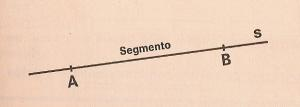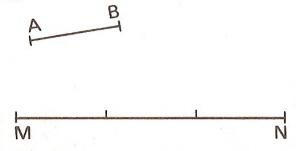Segments to printName: ______________________________________  Subject: _______________________ Date: _______

Write the missing word

1. The ray

The point P divides the line into two opposite rays. The point P is the origin of the two rays.2. The segment

Points A and B determine a portion of the line s that is called segment.

 The s is...... Points A and B form... The point A forms......3. Sum of segments

The segments can be added. We must put them on a line one behind the other.

In this case the segment AB + CD = AD

 The segment AD = The segment AB = The segment CD =4. Subtraction of segments

If we have the segment MN and we want subtract the segment PQ, we will form in a way that they start at the same point M. The segment QN is the difference.

 The segment QN = The Segment MN = The segment MQ =5. Multiplication of segments

To multiply the segment AB by 3, we will take a line and we will put three times that segment one after the other.

 The Segment MN = The segment AB =6. Consecutive and concatenated segments

The segments AB and BC are not on the same line and they are called concatenated.

The segments PQ and QR are called consecutive because they are in the same line.

Answers to these exercises:

 The segments PQ and QR are...... The segments AB and BC are...... The segments BC and AB are...... The segments QR and PQ are......7. Draw parallel lines

We see two ways to draw parallel lines with a triangle and a ruler.

In the first case they are the lines a, b and c. In the second case, they are the lines m, n and p.

| Educational applications | Mathematics |
In Spanish  | Interactive

«Arturo Ramo GarcĒa.-Record of intellectual property of Teruel (Spain) No 141, of 29-IX-1999
Plaza Playa de Aro, 3, 1║ DO 44002-TERUEL